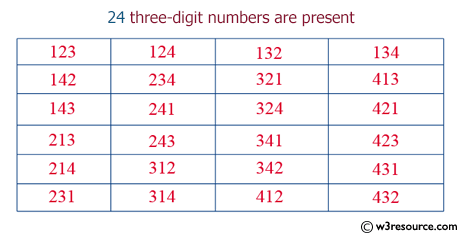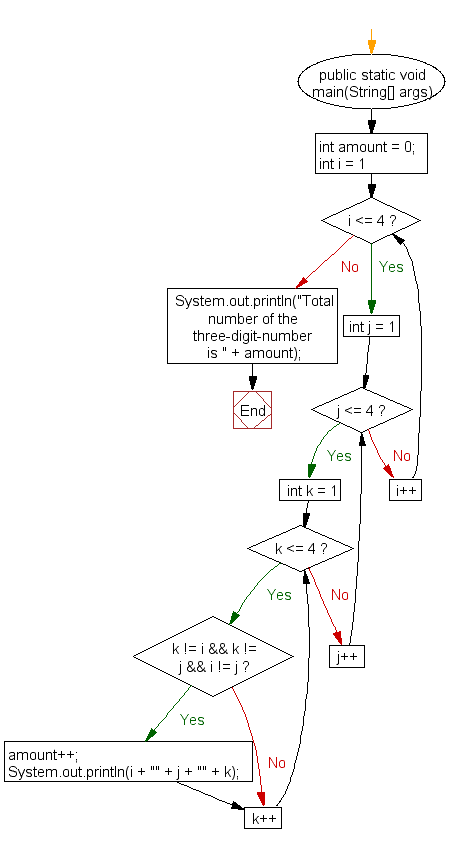﻿ Java: Display unique three digit numbers using 1, 2, 3, 4# Java Exercises: Create and display unique three-digit number using 1, 2, 3, 4. Also count how many three-digit numbers are there

## Java Basic: Exercise-39 with Solution

Write a Java program to create and display unique three-digit number using 1, 2, 3, 4. Also count how many three-digit numbers are there.

Pictorial Presentation:Sample Solution:

Java Code:

``````import java.util.Scanner;

public class Exercise39 {

public static void main(String[] args) {
int amount = 0;
for(int i = 1; i <= 4; i++){
for(int j = 1; j <= 4; j++){
for(int k = 1; k <= 4; k++){
if(k != i && k != j && i != j){
amount++;
System.out.println(i + "" + j + "" + k);
}
}
}
}
System.out.println("Total number of the three-digit-number is " + amount);
}
}
```
```

Sample Output:

```123
124
132
134
142
143
213
214
231
234
241
------
431
432
Total number of the three-digit-number is 24
```

Flowchart:Java Code Editor:

What is the difficulty level of this exercise?

Test your Programming skills with w3resource's quiz.

﻿

## Java: Tips of the Day

countOccurrences

Counts the occurrences of a value in an array.

Use Arrays.stream().filter().count() to count total number of values that equals the specified value.

```public static long countOccurrences(int[] numbers, int value) {
return Arrays.stream(numbers)
.filter(number -> number == value)
.count();
}
```

Ref: https://bit.ly/3kCAgLb